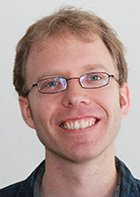# Prof. Dr. Wolfgang Steimle

Vorsitzender des Prüfungsausschusses Mathematik - Erasmusbeauftragter am Institut der Mathematik
Differentialgeometrie
 Telefon: +49 821 598 - 2208 E-Mail: wolfgang.steimle@math.uni-augsburg.de Raum: 3020 (L1) Sprechzeiten: nach Vereinbarung Adresse: Universitätsstraße 14, 86159 Augsburg## Research Interests

• Classification and automorphisms of manifolds
• Algebraic K- and L-theory
• Positive scalar curvature

## Publications

1. (with D. Crowley and T. Schick) The derivative map for diffeomorphism of disks: An example, 2020. To appear in Geom. Topol.
2. (with B. Calmès, E. Dotto, Y. Harpaz, F. Hebestreit, M. Land, K. Moi, D. Nardin and T. Nikolaus) Hermitian K-theory for stable infinity-categories I: Foundations, online first in Selecta Math. (2022).
3. An additivity theorem for cobordism categories, Algebr. Geom. Topol. 21 (2021), pp. 604-646.
4. (with G. Raptis) On the h-cobordism category. I, Int. Math. Res. Not. 23 (2021), pp. 18118-18148.
5. (with G. Raptis) Topological manifold bundles and the A-theory assembly map, Proc. Am. Math. Soc. 148 (2020), pp. 2787-2799.
6. (with D. Crowley and Th. Schick) Harmonic spinors and metrics of positive scalar curvature via the Gromoll filtration and Toda brackets, J. Topol. 11,4 (2018), pp. 1077-1099.
7. (with G. Raptis) A cobordism model for Waldhausen K-theory, J. Lond. Math. Soc. 99,2 (2018), pp. 516-534.
8. Degeneracies in quasi-categories, J. Homotopy Relat. Struct. 13,4 (2018), pp. 703-714.
9. (with F.T. Farrell and W. Lück) Approximately fibering a manifold over an aspherical one, Math. Ann. 370,1-2 (2018), pp. 669–726.
10. (with G. Raptis) Parametrized cobordism categories and the Dwyer-Weiss-Williams index theorem, J. Topol. 10,3 (2017), pp. 700-719.
11. (with W. Lück) Splitting the relative assembly map, Nil-terms and involutions, Ann. K-theory 1,4 (2016), pp. 339–377.
12. (with W. Lück) A twisted Bass-Heller-Swan decomposition for the non-connective K-theory of additive categories, Forum Math. 28,1 (2016), pp. 129-174.
13. (with B. Hanke and Th. Schick) The space of metrics of positive scalar curvature, Pub. Math. IHÉS 120,1 (2014), pp. 335–367.
14. (with W. Lück) Non-connective K- and Nil-spectra of additive categories, Contemp. Math. 617 (2014), pp. 205-236.
15. (with G. Raptis) On the map of Bökstedt-Madsen from the cobordism category to A-theory, Alg. Geom. Topol. 14,1 (2014), pp. 299-347.
16. Obstructions to stably fibering manifolds, Geom. Topol. 16,3 (2012), pp. 1691-1724.
17. Higher Whitehead torsion and the geometric assembly map, J. Topol. 5,3 (2012), pp. 529-574.
18. (with F.T. Farrell and W. Lück) Obstructions to fibering a manifold, Geom. Dedic. 148,1 (2010), pp. 35-69.